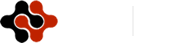International Journal of Electronic Engineering and Computer Science, Vol. 1, No. 1, August 2016 Publish Date: Aug. 16, 2016 Pages: 35-39

Cumulative Analysis of Measurement Processes and a Correcting Filtration

Mehdiyeva A. M.*

Control and System Engineering Department, Azerbaijan State Oil and Industry University, Baku, Azerbaijan

Abstract

The offered systematic approach to creation of information-measuring systems of considered parameters consists of cumulative analysis of measurement processes and a correcting filtration for the purpose of achievement of the balanced metrological, structurally-algorithmic and functional efficiency indicators of developed means.

Keywords

Non-sinusoidal Signal, Digital Measurements, Error of Measurement, Measurement Processes, Correcting Filtration

Contents

1. Introduction

With an ever-growing need for information-measuring systems (IMS), the variety of their performances and applications with increasing requirements for quality and efficiency of existing and newly developed systems, the task of designing and developing the theoretical, methodological and practical aspects of the creation of high-performance IMS is of particular relevance.

Efficiency is a comprehensive indicator of the quality of IMS, reflecting the system's ability to provide given the technical, economical and operational performance at the performance of the measurement, information and logic functions within the automation of service facilities.

On the theoretical level, it is of paramount importance to optimize the metabolic processes (receipt, transformation, transmission, processing and reporting) measurement information to IMS to increase, above all, the metrological quality of the results of measurement, control, diagnostics and identification within existing techno-economic constraints . With regard to the IMS solution of optimization problems considerably difficulties, which in most cases are associated with deficiency of a priori information about the properties and characteristics of automation object, the measured values, interfering factors, intermediate and final results of solving the basic system functions and tasks, as well as the functional units of measurement and computer subsystems. In the synthesis of the optimal IMS and their subsystems working in dynamic mode, the problem of overcoming a priori uncertainty is further compounded. Now, when high precision dynamic measurement becomes widespread, this problem is of interest for further development of information and measurement technology in general.

Control parameters and diagnostics of power facilities is an actual problem of increasing the efficiency of the fuel and energy complex of the country. This is because the quality of the electricity generated by power units, and the amount of electricity consumed by power plants, ultimately determine the performance indicators of the whole complex.

2. Statement of the Problem

Currently, in a given direction, intensive research and development. Unfortunately, however, a systematic approach to the creation of means of control parameters diagnostics of power facilities available.

In this article an attempt is made to develop a systematic approach to creating MIS reporting purposes. At the same time as the object considered asynchronous motors that are the foundation of most electric drives, which account for about 40% of electricity consumed in the world .

Any measured value, for example, the voltage is a sine wave signal. As a part of this signal has only one harmonic. As a result, the measurement of an analog value, we obtain a non-sinusoidal signal. It is a sinusoid having a composition further odd harmonics. The cause of these harmonics are noise and interference imposed on the main signal and errors it. Therefore, the measured signals, in most cases (80%) are non-sinusoidal [3,4].

Curves of instantaneous values of continuous signals are most informative, but not always foreseeable. Therefore, for the management and analysis of controlled processes and objects of great importance is the definition of real-time integrated signal parameters (IPS). Recent characterize the total amount of matter and energy to be made and received in the production for a certain period of time, regime indicators and parameters of the object, is the average of the measured values, etc. The specifics of determining the IPS digital methods and tools is to perform discrete averaging (DA) or digital integration (DI) values, continually changing over time.

Among the digital measurement methods the IRS is widely used so-called method of digital processing of the results of direct measurements of instantaneous values of the signal during averaging (integration). In recent years, interest in this technique has increased considerably, due to the possibility of the introduction of computing power-measuring channels for solving this kind of measurement tasks. This problem becomes even more complicated when the digital measurements IPS electrical signals of complex shape (non-sinusoidal signals). Nevertheless, the well-known advantages of electrical methods for monitoring and measuring physical variables contribute to more widespread primary transmitters of information to the output signals in the form of fixed and variable currents and voltages.

AC signals are more informative, and in some cases are the only possible form of reception measurement information, particularly in objects and generate electrical power conversion.

In the transition from continuous integration to the numerical algorithms it is necessary to draw attention to the fact that almost all the algorithms used by the operator control. To perform the control class of continuous functions using the quadrature formula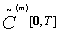of the form: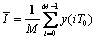,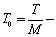where in the sampling step time.

The article presents a systematic approach to the design of MIS for monitoring parameters and assess the current state of electric motors.

As is well known , motors diagnosis can be performed by measuring and processing the values of the currents and power idling and short-circuit. On how accurately make measurements of these parameters depends on the accuracy of control and diagnostics.

Full power is determined by the voltage U in the network and the current I, through the lens: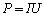.                                  (1)

If the measurement of any of the parameters that determine the power error will be introduced (and it is always present), the result is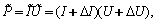(2)

where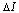- the resulting error in current measurement - resulting measurement error voltage.

In view of the result of measurement errors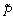in power will differ from the true value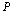by: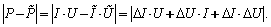(3)

During the measurement of current and voltage harmonic signal it is useful noisy, i.e. it imposed as a result of measurement of high-frequency noise (interference). As a result, pure harmonic signal is anharmonic. Moreover, in actual circuit determines the presence of additional harmonics non-sinusoidality also themselves the measurement signals u(t) and i(t).

3. Parameter Estimation Signals

Consider the case where a non-sinusoidal signal interacts with random noise centered, in particular, we find out what is the range of products of these signals and how he modified after applying the filter of discrete averaging required for digital measurements of integral characteristics of AC signals. The output of the filter is defined as follows :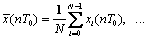(4)

where N - number of averaged samples, T0 - a step in the sampling time.

As an initial non-sinusoidal signal, consider the sine wave with a frequency of 50Hz with superimposed on it 13 odd harmonics of the form: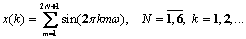(5)

where w - the fundamental frequency of the signal to the - number of discrete samples, m - number of harmonics.

As the random noise centered consider a random signal uniformly distributed on the interval [0,1] and generated a built-in Matlab rand. The spectra of the original signal are calculated in the software Matlab environment using a fast Fourier transform - function fft.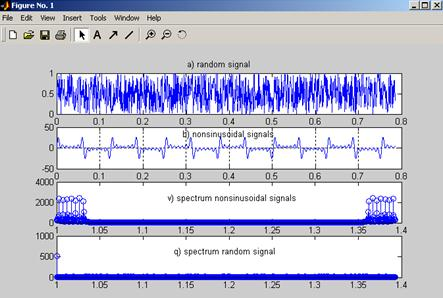Fig. 1. Initial signals and their spectra.

In accordance with the expression (3) (assuming one of signals- it, and the other - U), we find the work of the original signals. From the theory of spectral analysis is well known , as a result of multiplying the waveform of the corresponding spectra are stacked. The resulting signal and the total spectrum depicted in Figure 2. As seen from Figure 2 (b), the resultant spectrum contains in its structure a large amount of additional harmonics as a consequence of the superposition of different frequencies in the random signal.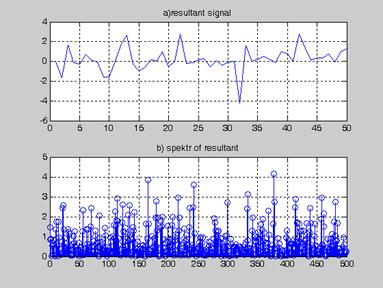Fig. 2. The resulting signal and its spectrum.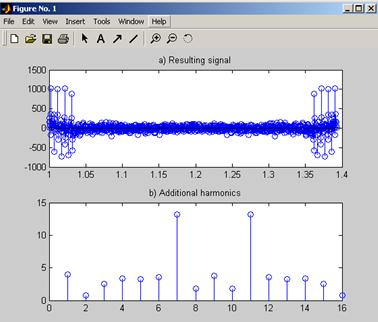Fig. 3. Signal and the filter output spectrum.

To suppress harmonics additional skip resultant signal through digital averaging filter. For this, the entire time interval is divided into subintervals and each of them find the mean value of samples of the resultant signal in the form: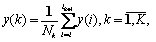(6)

where Nk - the number of samples in each interval, lk and lk + 1 - boundaries of the intervals, K - the number of sub-intervals.

To investigate the harmonic content of the received signal spectrum we find the output of the filter (Figure 3).

The calculations presented in Figure 3 show that the spectrum is significantly reduced the impact of the additional harmonics. Their amplitude is significantly (by several orders) decreased, that is, was the cleansing of the spectrum of side components. Therefore, with respect to the non-sinusoidal signals available to suppress random noise in them enough to pass through the measurement result averaging digital filter, and only then to use the corrected signal as a parameter for control and diagnostics. A similar calculation was made by us in relation to the non-sinusoidal signal and bias about 2 described function of the form [6-11]: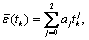(7)

where tk - discrete samples bias, AJ - coefficients of the polynomial describing the slowly changing error.

The signal spectrum by filtering averaging digital filter is significantly decreased. Comparative analysis of the results of the experiment with the random and systematic errors shows that the best corrected systematic error.

Consequently, the construction of IMS for monitoring and diagnosis of pre-measured control parameters should be adjusted by applying thereto digital averaging filter, and only then on the basis of the adjusted results to monitor and diagnose . According to the above procedure also covers other typical errors due to random variations in the points of reference of the instantaneous values of the measurement signals (the error of dating samples), the effect of quantization levels in the analog-to-digital conversion signal samples (quantization noise) and digital (round off noise).

4. Conclusion

Thus, the proposed systematic approach to the creation of IMS reporting purpose is comprehensive analysis of the processes of measurement and correction filter in order to achieve a balanced scorecard metrology, structural and functional efficiency of algorithmic tools developed.

References

1. Telman Aliyev. Robust Technology with Analysis of Interference in Signal Processing. New-York: Kluwer, 2003. 199 p.
2. Arutyunov P.A. Theory and Application of algorithmic measurements. M.: Energoatomizdat, 1999, 256 p.
3. Goldberg O.D., Abdullayev I.M., Abiyev A.N. Automation of the control parameters and diagnostics of induction motors. M.: Energoatomizdat, 1997, 160 p.
4. Abdullayev I.M., Allahverdiyeva N.R. Correction filter in the means of measurement. Baku: Chashiogli 2005, 184 p.
5. Sergienko A.B. Digital signal processing. SPb.: Peter, 2005, 604 p.
6. L.A.Rams. Level quantization and sampling time in digital control systems. M.: Energoatomizdat, 1999, 304 p.
7. Bruchanov V. A. Methods of improving the accuracy of measurements in industry. M.: Publishing House of Standards, 1991. 108 p.
8. W.Stewen Smit. Digital Signal Processing. California Technical Publishing. ISBN 0-9660176-3-3. 1997. 630 p.
9. Sensors EXPO, September 14-16, 1999, Cleverland, Ohio USA.
10. Pbentley J. Principles of Measurements Systems.N.Y.:Longman Inc. Construction Press, 1993. 400p.
11. Mehdiyeva A. M. et al. Conversion and initial processing errors of measurement results. American Journal of Circuits, Systems and Signal Processing. Vol. 1, No 3, 2015, pp. 56-59.
12. Mehdiyeva A.M., Mehdizadə E.K. Information-measurement system development for controlling of parameters of measurement.Global Standart Journal. Vol 1(2): 030-033, March 2014.

 Contents 1. 2. 3. 4.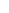600 ATLANTIC AVE, BOSTON,
MA 02210, USA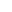+001-6179630233
JournalsJournal ListsAuthor GuidelinesPayment InformationSubmit a ManuscriptOpen Special IssuesJoin as Editorial MembersJoin as ReviewersPrivacy NoticeTerms of ConditionsHelp Center